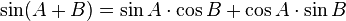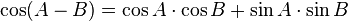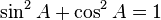## Trigonometric formulae

The trigonometric functions are defined with the aid of the unit circle as follows:The perhaps most important trigonometric formulas from which almost all other trigonometric formulas can be derived are the angle transformation formulas:These formulae can be derived by computing the distance between two points on the unit circle  with the distanceformula and with the cosinetheorem and then equating them to each other.
And by direct application of the Pythagorean theorem on the unit circle one obtains the important relationconnecting sine and cosine.

Annonser## Om mattelararen

Licentiate of Philosophy in atomic Physics Master of Science in Physics
Det här inlägget postades i Geometri, Gymnasiematematik(high school math), matematik 4 och har märkts med etiketterna , . Bokmärk permalänken.